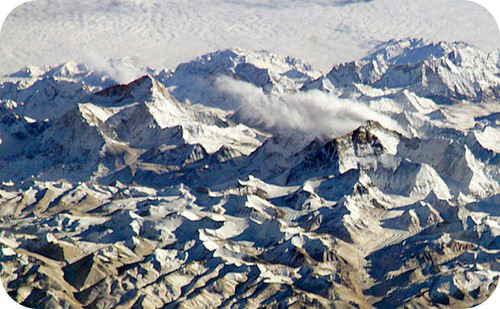# 11.12: Boiling

$$\newcommand{\vecs}{\overset { \rightharpoonup} {\mathbf{#1}} }$$ $$\newcommand{\vecd}{\overset{-\!-\!\rightharpoonup}{\vphantom{a}\smash {#1}}}$$$$\newcommand{\id}{\mathrm{id}}$$ $$\newcommand{\Span}{\mathrm{span}}$$ $$\newcommand{\kernel}{\mathrm{null}\,}$$ $$\newcommand{\range}{\mathrm{range}\,}$$ $$\newcommand{\RealPart}{\mathrm{Re}}$$ $$\newcommand{\ImaginaryPart}{\mathrm{Im}}$$ $$\newcommand{\Argument}{\mathrm{Arg}}$$ $$\newcommand{\norm}{\| #1 \|}$$ $$\newcommand{\inner}{\langle #1, #2 \rangle}$$ $$\newcommand{\Span}{\mathrm{span}}$$ $$\newcommand{\id}{\mathrm{id}}$$ $$\newcommand{\Span}{\mathrm{span}}$$ $$\newcommand{\kernel}{\mathrm{null}\,}$$ $$\newcommand{\range}{\mathrm{range}\,}$$ $$\newcommand{\RealPart}{\mathrm{Re}}$$ $$\newcommand{\ImaginaryPart}{\mathrm{Im}}$$ $$\newcommand{\Argument}{\mathrm{Arg}}$$ $$\newcommand{\norm}{\| #1 \|}$$ $$\newcommand{\inner}{\langle #1, #2 \rangle}$$ $$\newcommand{\Span}{\mathrm{span}}$$$$\newcommand{\AA}{\unicode[.8,0]{x212B}}$$Figure $$\PageIndex{1}$$ (Credit: Courtesy of the Earth Observatory/NASA; Source: Commons Wikimedia, Mount Everest(opens in new window) [commons.wikimedia.org]; License: Public Domain)

### Why is it harder to breathe at the top of Mount Everest than at the bottom?

At 29,029 feet $$\left( 8848 \: \text{m} \right)$$, Mount Everest in the Himalayan range, on the border between China and Nepal, is the highest point on the earth. Its altitude presents many practical problems to climbers. The oxygen content of the air is much lower than at sea level, making it necessary to bring oxygen tanks along (although a few climbers have reached the peak without oxygen). One other problem is that of boiling water for cooking food. Although water boils at $$100^\text{o} \text{C}$$ at sea level, the boiling point on top of Mount Everest is only about $$70^\text{o} \text{C}$$. This difference makes it very difficult to get a decent cup of tea (which definitely frustrates some British climbers).

## Boiling Point

As a liquid is heated, the average kinetic energy of its particles increases. The rate of evaporation increases as more and more molecules are able to escape the liquid's surface into the vapor phase. Eventually a point is reached when the molecules all throughout the liquid have enough kinetic energy to vaporize. At this point, the liquid begins to boil. The boiling point is the temperature at which the vapor pressure of a liquid is equal to the external pressure. The figure below illustrates the boiling of liquid.Figure $$\PageIndex{2}$$: Comparison between evaporation and boiling. (Credit: Christopher Auyeung; Source: CK-12 Foundation; License: CC BY-NC 3.0(opens in new window))

In the picture on the left, the liquid is below its boiling point, yet some of the liquid evaporates. On the right, the temperature has been increased until bubbles begin to form in the body of the liquid. When the vapor pressure inside the bubbles is equal to the external atmospheric pressure, the bubbles rise to the surface of the liquid and burst. The temperature at which this process occurs is the boiling point of the liquid.

The normal boiling point is the temperature at which the vapor pressure of the liquid is equal to standard pressure. Because atmospheric pressure can change based on location, the boiling point of a liquid changes with the external pressure. The normal boiling point is a constant because it is defined relative to the standard atmospheric pressure of $$760 \: \text{mm} \: \ce{Hg}$$ (or $$1 \: \text{atm}$$ or $$101.3 \: \text{kPa}$$).Figure $$\PageIndex{3}$$: Influence of altitude on the boiling point of water. (Credit: Joy Sheng; Source: CK-12 Foundation; License: CC BY-NC 3.0(opens in new window))

## Summary

• The boiling point is the temperature at which the vapor pressure of a liquid is equal to the external pressure.
• As the altitude increases, the boiling point decreases.

## Review

1. What happens when a liquid is heated?
2. What is the boiling point?
3. What is the pressure at which the normal boiling point is determined?
4. Are the boiling point and normal boiling point always the same value, sometimes the same value, or never the same value? Explain your answer.

## Explore More

Use the video below to answer the following questions:

1. The boiling point of water is 100°C. What is an example of liquid that boils a temperature higher than the boiling point of water?
2. What is an example of liquid that boiling point is below room temperature?
3. What phase change is the opposite of boiling?

This page titled 11.12: Boiling is shared under a CK-12 license and was authored, remixed, and/or curated by CK-12 Foundation via source content that was edited to the style and standards of the LibreTexts platform; a detailed edit history is available upon request.